### Coefficient of Variation

If you specify the CV option in the TABLES statement, PROC SURVEYFREQ computes the coefficients of variation for the proportion estimates in the frequency and crosstabulation tables. The coefficient of variation is the ratio of the standard error to the estimate.

For the proportion in table cell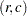, the coefficient of variation is computed as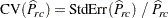where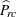is the estimate of the proportion in table celland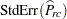is the standard error of the estimate. The coefficients of variation for row proportions and column proportions are computed similarly.

If you specify the CVWT option in the TABLES statement, PROC SURVEYFREQ computes the coefficients of variation for the weighted frequencies (estimated totals) in the crosstabulation tables. For the total in table cell, the coefficient of variation is computed as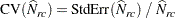where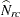is the estimate of the total in table celland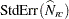is the standard error of the estimate. The coefficients of variation for row totals, column totals, and the overall total are computed similarly.